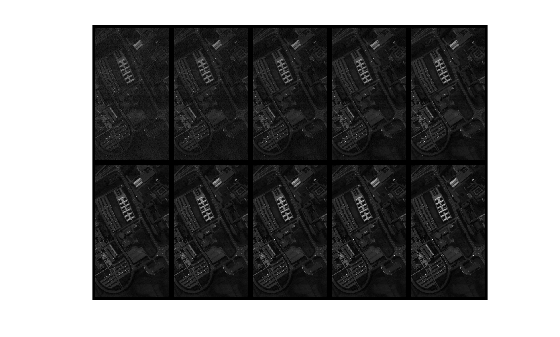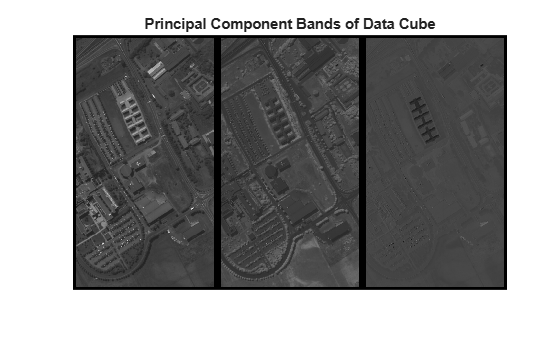Main Content

# hyperpca

Principal component analysis of hyperspectral data

## Syntax

``outputDataCube = hyperpca(inputData,numComponents)``
``[outputDataCube,coeff] = hyperpca(___)``
``[outputDataCube,coeff,var] = hyperpca(___)``
``[___] = hyperpca(___,Name,Value)``

## Description

example

````outputDataCube = hyperpca(inputData,numComponents)` computes the specified number of principal components from the spectral bands of the hyperspectral data cube. The function returns a new data cube that contains the principal component bands. The number of spectral bands in the output data cube is equal to the number of specified principal components `numComponents`. To achieve spectral dimensionality reduction, the specified number of principal components must be less than the number of spectral bands in the hyperspectral data cube `inputData`.```
````[outputDataCube,coeff] = hyperpca(___)` also returns the principal component coefficients estimated across the spectral dimension of the hyperspectral data cube.```

example

````[outputDataCube,coeff,var] = hyperpca(___)` returns the percentage of variance retained by the principal component bands in addition to the output arguments mention in the previous syntaxes.```

example

````[___] = hyperpca(___,Name,Value)` specifies the principal component analysis (PCA) method and additional options by using the name-value pair arguments. NoteThis function requires the Image Processing Toolbox™ Hyperspectral Imaging Library. You can install the Image Processing Toolbox Hyperspectral Imaging Library from Add-On Explorer. For more information about installing add-ons, see Get and Manage Add-Ons. ```

## Examples

collapse all

Read a hyperspectral data into the workspace.

`hcube = hypercube('paviaU.dat');`

Compute the principal component bands of the hyperspectral data cube. Specify the number of principal components to extract as 10. By default, the function uses the singular value decomposition (SVD) method for extracting principal components.

`reducedDataCube = hyperpca(hcube,10);`

Display the first 10 spectral bands in input data cube.

```figure montage(hcube.DataCube(:,:,1:10),'BorderSize',[10 10],'Size',[2 5],'DisplayRange',[]);```For the purpose of visualization, rescale the principal component values to lie in the range [0, 1]. Display all the principal component bands extracted from the data cube.

```figure rescalePC = rescale(reducedDataCube,0,1); montage(rescalePC,'BorderSize',[10 10],'Size',[2 5]); title('Principal Component Bands of Data Cube')```Read a hyperspectral data into the workspace.

`hcube = hypercube('paviaU.dat');`

Perform PCA of input data cube using Eigen value decomposition. Specify the number of principal components to extract as 3. Derive the principal component (PC) bands, coefficients, and retained variance.

`[outputDataCube,coeff,var] = hyperpca(hcube,3,'Method','Eig');`

For the purpose of visualization, rescale the principal component values to lie in the range [0, 1]. Display all the principal component bands extracted from the data cube.

```figure rescalePC = rescale(outputDataCube,0,1); montage(rescalePC,'BorderSize',[10 10],'Size',[1 3]); title('Principal Component Bands of Data Cube')```Plot the principal component coefficients and display the percentage of variance retained by each of the principal components. The summation of retained variance values imply that almost 99% of the information in input hyperspectral data is captured by the 3 principal components.

```figure plot(hcube.Wavelength,coeff); legend(['PC1';'PC2';'PC3'],'Location','SouthEast') text(430,0.19,'Retained variance'); text(430,0.17,['PC1: ' num2str(var(1))]) text(430,0.15,['PC2: ' num2str(var(2))]) text(430,0.13,['PC3: ' num2str(var(3))]) xlabel('Wavelength') ylabel('PC Coefficients')```## Input Arguments

collapse all

Input hyperspectral data, specified as a 3-D numeric array that represent the hyperspectral data cube of size M-by-N-by-C or `hypercube` object. If the input is a `hypercube` object, the function reads the data from the `DataCube` property of the object. The hyperspectral data cube must be real and non-sparse.

Data Types: `single` | `double` | `int8` | `int16` | `int32` | `int64` | `uint8` | `uint16` | `uint32` | `uint64`

Number of principal component bands to extract from the data cube, specified as a positive integer scalar. The value must be less than or equal to the number of spectral bands in the input data cube.

Data Types: `single` | `double` | `int8` | `int16` | `int32` | `int64` | `uint8` | `uint16` | `uint32` | `uint64`

### Name-Value Pair Arguments

Specify optional comma-separated pairs of `Name,Value` arguments. `Name` is the argument name and `Value` is the corresponding value. `Name` must appear inside quotes. You can specify several name and value pair arguments in any order as `Name1,Value1,...,NameN,ValueN`.

Example: `hyperpca(hcube,10,'Method','eig')`

Method for PCA, specified as one of these values:

• '`svd`' — To derive principal components by using the singular value decomposition method.

• '`eig`' — To derive principal components by using the eigen value decomposition method.

Data Types: `char` | `string`

Indicator for mean centering spectral bands, specified as one of these values:

• `true` or 1 — To center each spectral bands in the input data cube by subtracting the mean of spectral bands before computing the principal component bands.

• `false` or 0 — To compute principal component bands without mean centering the spectral bands in the input data cube.

Data Types: `logical`

## Output Arguments

collapse all

PCA transformed data cube, returned as a 3-D numeric array of size M-by-N-by-numComponents. The spatial dimension of the output data cube is same as that of the input data cube. The spectral dimension of the output data cube is equal to the specified number of principal components `numComponents`.

If the input data type is double, the output data type is also double. Otherwise, the output data type is single.

Data Types: `single` | `double`

Principal component coefficients, returned as a matrix of size C-by-numComponents. C is the number of spectral bands in the input data cube. Each column of `coeff` contains the coefficients for one principal component. The columns are in the order of descending component variance.

If the input data type is double, the data type of `coeff` is also double. Otherwise, the data type is single.

Data Types: `single` | `double`

Variance retained by each principal component, returned as a vector of length equal to `numComponents`. The retained variance specifies the total percentage of variance explained by each principal component.

If the input data type is double, the data type of `var` is also double. Otherwise, the data type is single.

Data Types: `single` | `double`

## See Also

Introduced in R2020a

## Support

#### Introducing Deep Learning with MATLAB

Download ebook